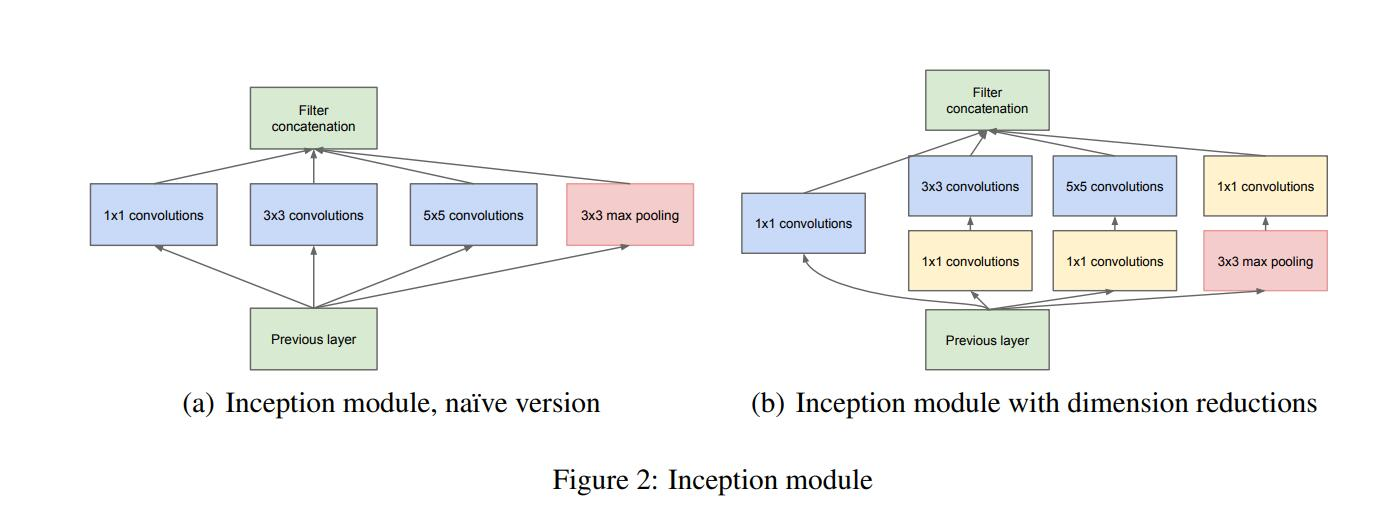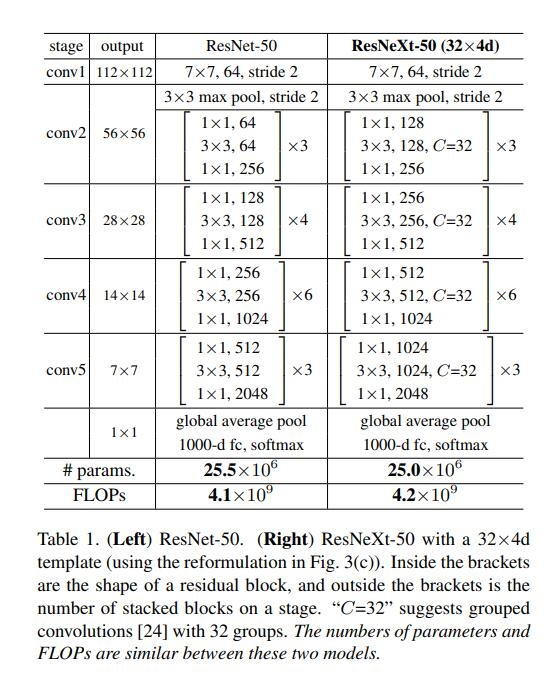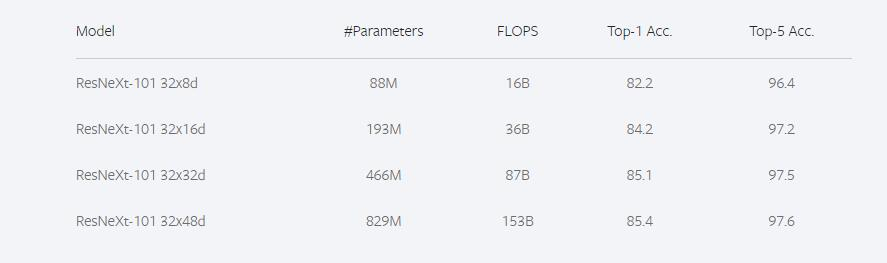# ResNeXt¶

## 1. 模型介绍¶

ResNeXt是由何凯明团队在2017年CVPR会议上提出来的新型图像分类网络。ResNeXt是ResNet的升级版，在ResNet的基础上，引入了cardinality的概念，类似于ResNet，ResNeXt也有ResNeXt-50，ResNeXt-101的版本。那么相较于ResNet，ResNeXt的创新点在哪里？既然是分类网络，那么在ImageNet数据集上的指标相较于ResNet有何变化？之后的ResNeXt_WSL又是什么东西？下面我和大家一起分享一下这些知识。

## 2. 模型结构¶

ResNeXt正是借鉴了这种“分割-变换-聚合”的策略，但用相同的拓扑结构组建ResNeXt模块。每个结构都是相同的卷积核，保持了结构的简洁，使得模型在编程上更方便更容易，而InceptionNet则需要更为复杂的设计。## 3. 模型实现¶

```class ConvBNLayer(nn.Layer):
def __init__(self, num_channels, num_filters, filter_size, stride=1,
groups=1, act=None, name=None, data_format="NCHW"
):
super(ConvBNLayer, self).__init__()
self._conv = Conv2D(
in_channels=num_channels, out_channels=num_filters,
kernel_size=filter_size, stride=stride,
padding=(filter_size - 1) // 2, groups=groups,
weight_attr=ParamAttr(name=name + "_weights"), bias_attr=False,
data_format=data_format
)
if name == "conv1":
bn_name = "bn_" + name
else:
bn_name = "bn" + name[3:]
self._batch_norm = BatchNorm(
num_filters, act=act, param_attr=ParamAttr(name=bn_name + '_scale'),
bias_attr=ParamAttr(bn_name + '_offset'), moving_mean_name=bn_name + '_mean',
moving_variance_name=bn_name + '_variance', data_layout=data_format
)

def forward(self, inputs):
y = self._conv(inputs)
y = self._batch_norm(y)
return y

class BottleneckBlock(nn.Layer):
def __init__(self, num_channels, num_filters, stride, cardinality, shortcut=True,
name=None, data_format="NCHW"
):
super(BottleneckBlock, self).__init__()
self.conv0 = ConvBNLayer(num_channels=num_channels, num_filters=num_filters,
filter_size=1, act='relu', name=name + "_branch2a",
data_format=data_format
)
self.conv1 = ConvBNLayer(
num_channels=num_filters, num_filters=num_filters,
filter_size=3, groups=cardinality,
stride=stride, act='relu', name=name + "_branch2b",
data_format=data_format
)

self.conv2 = ConvBNLayer(
num_channels=num_filters,
num_filters=num_filters * 2 if cardinality == 32 else num_filters,
filter_size=1, act=None,
name=name + "_branch2c",
data_format=data_format
)

if not shortcut:
self.short = ConvBNLayer(
num_channels=num_channels, num_filters=num_filters * 2
if cardinality == 32 else num_filters,
filter_size=1, stride=stride,
name=name + "_branch1", data_format=data_format
)

self.shortcut = shortcut

def forward(self, inputs):
y = self.conv0(inputs)
conv1 = self.conv1(y)
conv2 = self.conv2(conv1)

if self.shortcut:
short = inputs
else:
short = self.short(inputs)

y = F.relu(y)
return y
```

## 4. 模型特点¶

1. ResNeXt通过控制cardinality的数量，使得ResNeXt的参数量和GFLOPs与ResNet几乎相同。

2. 通过cardinality的分支结构，为网络提供更多的非线性，从而获得更精确的分类效果。

## 5. 模型指标¶ResNeXt101_32×48d_WSL有8亿+的参数，是通过弱监督学习预训练的方法在Instagram数据集上训练，然后用ImageNet数据集做微调，Instagram有9.4亿张图片，没有经过特别的标注，只带着用户自己加的话题标签。 ResNeXt_WSL与ResNeXt是一样的结构，只是训练方式有所改变。下图是ResNeXt_WSL的训练效果。ResNet

ResNeXt

```